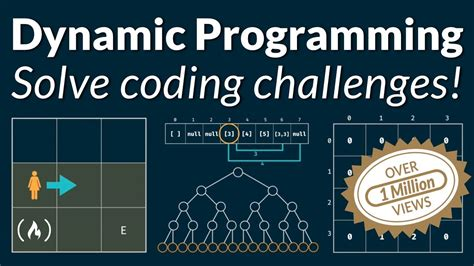# Dynamic Programming: A Powerful Tool for Solving ProblemsDynamic Programming is a powerful tool for solving problems. It is an algorithmic technique used to solve complex problems by breaking them down into smaller subproblems and then combining the solutions of those subproblems to obtain the solution of the original problem. Dynamic programming can be applied to many different types of problems, including optimization, scheduling, resource allocation, and game theory.

The main idea behind dynamic programming is that it allows us to break down a complex problem into simpler subproblems. By doing this, we can reduce the complexity of the problem and make it easier to solve. We can also use dynamic programming to find optimal solutions to certain problems. This means that we can find the best possible solution to a given problem in the least amount of time.

To understand how dynamic programming works, let’s look at an example. Suppose you are trying to find the shortest path between two points on a map. You could try to solve this problem using brute force, which would involve checking every single route from one point to another. However, this approach would take too long and may not even yield the correct answer. Instead, you could use dynamic programming to break down the problem into smaller subproblems. For instance, you could start by finding the shortest paths between each pair of adjacent points on the map. Then, you could combine these shorter paths to form the overall shortest path between the two points.

Dynamic programming can also be used to solve problems involving decision making. In such cases, dynamic programming helps us determine the best course of action based on the available information. For example, if you were trying to decide whether or not to invest in a particular stock, you could use dynamic programming to evaluate all the potential outcomes and choose the option with the highest expected return.

Finally, dynamic programming can be used to solve problems related to game theory. Game theory involves analyzing the strategies of players in order to determine the most advantageous outcome for each player. By using dynamic programming, we can analyze the various strategies of each player and determine the best strategy for each situation.

In conclusion, dynamic programming is a powerful tool for solving problems. It can be used to optimize solutions, make decisions, and analyze game theory scenarios. With its ability to break down complex problems into simpler subproblems, dynamic programming can help us find the best possible solutions to any given problem.